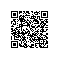# 新手学习之查看ORACLE 数据库表空间和数据表的大小

1.列如我们查看特定表大小占用表空间大小

select sum(bytes)/1024/1024 Mbytes  from user_segments where segment_type='TABLE' and segment_name='TEST01';

select segment_name, bytes from user_segments where segment_type = 'TABLE';

2.查看表当前使用的空间

analyze table test01 compute statistics; 分析表

select num_rows * avg_row_len  from user_tables  where table_name = 'TEST01'; 查询表

select * from user_tablespaces

select tablespace_name,sum(nvl(bytes,0)) from dba_free_space group by tablespace_name

Nvl(a,b)，就是用户判断a的值，如果查询的结果a的值为null，则返回b的值，如果a的值不为null，则返回a的值

select tablespace_name,sum(bytes) from dba_data_files group by tablespace_name;

select tablespace_name,contents,extent_management from dba_tablespaces

select

a.a1  tab_name,   （表空间名称）

c.c2  tab_type,（表类型）

c.c3  table_management, （表管理）

b.b2/1024/1024  tab_space_M,　　　（表空间大小，总）

a.a2/1024/1024  free_space_M,（剩余表空间大小）

(b.b2-a.a2)/1024/1024  USED_SPACE_M,（ 表使用大小)

substr((b.b2-a.a2)/b.b2*100,1,5)   use_ratio(表的利用率)

from

(select tablespace_name a1,sum(nvl(bytes,0)) a2 from dba_free_space group by tablespace_name)  a,

(select tablespace_name b1,sum(bytes) b2 from dba_data_files group by tablespace_name) b,

(select tablespace_name c1,contents c2,extent_management c3 from dba_tablespaces)  c

where a.a1=b.b1 and c.c1=b.b1;1.   查看当前库下所有表空间的free情况

select tablespace_name,sum(nvl(bytes,0)) from dba_free_space group by tablespace_name

2.   查看表空间所属的数据文件，测试环境下，一般都是一个表空间下只有一个数据文件，而在实际的环境中，一个表空间下有多个数据文件，而一个数据文件只能属于一个表空间

select file_name,tablespace_name from dba_data_files;

alter tablespace myspace add datafile '/opt/oracle/oradata/test05/mytb02.dbf' size 20M autoextend on next 2m maxsize 100m;

创建视图，查看file_nametablespace_name，如下

select

b.file_name       phy_file_name,     （物理文件名）

b.tablespace_name   tablespace_name,（表空间名）

b.bytes/1024/1024   tabspace_bytes,（表空间大小 MB

(b.bytes-sum(nvl(a.bytes,0)))/1024/1024   USEED_SPACE,（使用表空间大小）

substr((b.bytes-sum(nvl(a.bytes,0)))/(b.bytes) * 100,1,5)  use_ratio （利用率）

from dba_free_space a , dba_data_files b

where a.file_id=b.file_id

group by b.tablespace_name,b.file_name,b.bytes

order by b.tablespace_nameselect a.table_name,a.tablespace_name,b.file_name from user_tables a , dba_data_files b

where a.tablespace_name=b.tablespace_name and a.table_name='TEST01';

:查询和表空间的相关查询命令（知识点）

1.  查询默认的数据表空间和临时表空间

select property_name,property_value from database_properties where property_name in ('DEFAULT_PERMANENT_TABLESPACE',

'DEFAULT_TEMP_TABLESPACE');  （针对USER的默认数据表空间和临时表空间）

2.  更改数据库的默认表空间

Alter database  default  tablespace tablespace_name;(数据表空间）

Alter database  temporary tablespace tablespace_name;(临时数据表空间)

本文转自天真花语  51CTO博客，原文链接：http://blog.51cto.com/caibird/1285749，如需转载请自行联系原作者使用钉钉扫一扫加入圈子
+ 订阅